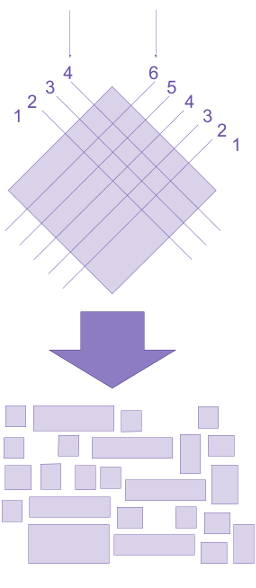# Commutativity as an artifact of notation

Instead of thinking of a commutative function $$f(x, y)$$ as a function that takes an ordered pair of inputs, we can think of $$f$$ as a function that takes an unordered bag of inputs, and therefore can’t depend on their order. On this interpretation, the fact that functions are always given inputs in a particular order is an artifact of our definitions, not a fundamental property of functions themselves. If we had notation for functions applied to arguments in no particular order, then commutative functions would be the norm, and non-commutative functions would require additional structure imposed on their inputs.

In a world of linear left-to-right notation, where $$f(x, y)$$ means “$f$ applied to $$x$$ first and $$y$$ second”, commutativity looks like a constraint. In an alternative world where functions are applied to their inputs in parallel, with none of them distinguished as “first” by default, commutativity is the natural state of affairs.

# Commutativity as symmetry in the output

Commutativity can be seen as a form of symmetry in the output of a binary function. Imagine a binary function as a physical mechanism of wheels and gears, that takes two inputs in along conveyer belts (one on the left, one on the right), manipulates those inputs (using mechanical sensors and manipulators and tools), and produces a result that is placed on an outgoing conveyer belt.

The output of a commutative function is symmetric in the way it relates to the inputs. Consider a function that takes two wooden blocks and glues them together. The function might manipulate them in a symmetric fashion (if both blocks are picked up simultaneously, and have glue applied simultaneously, and are pushed together simultaneously), but the output is not symmetric: If a red block comes in on the left and a blue block comes in on the right, then the resulting block is red on the left and blue on the right, and the function is not commutative (though it is associative).

By contrast, a function that mixes the red and blue together — producing, for example, the uniform color purple or the unordered set $$\{b, d, e, l, u, r\}$$ — would be commutative, because the way that the output relates to each input is independent of which side the input came in on.

A function is probably commutative if you can visualize the output itself as left/​right symmetric, even if the left input was very different from the right input. For example, we can use the following visualization to show that multiplication is commutative. Imagine a function that takes in two stacks of poker chips, one on the left conveyer belt and one on the right conveyer belt. The function has a square of wet plaster in the middle. On each conveyer belt, an arm removes chips from the stack of poker chips until the stack only has one chip left, and for each chip that is removed, a perpendicular cut is put in the plaster (as in the diagram below). The plaster is then allowed to set, and the plaster pieces are then shaken out into a big bag. A third arm removes plaster pieces from the bag one at a time, and adds a poker chip to the outgoing conveyer belt for each one. To visualize this, see the diagram below:If the input conveyer belts had 4 and 6 poker chips on them (respectively), then the output belt will have 24 chips on it (because 24 different chunks of plaster will have fallen into the bag), but the chips don’t necessarily come from any one particular side: The output relates to each input in a manner that doesn’t depend on which side they came in.

knows-requisite(Math 2): In mathematics, a symmetry is some structure on a set that is preserved under some transformation of that set. In our case, the structure is the value of the output, which is preserved under the transformation of changing which side the inputs come in on. Formally, let $$X^2$$ be the set of all pairs of two values from the set $$X;$$ each point in $$X^2$$ is a pair $$(x_1, x_2).$$ $$X^2$$ can be visualized as an $$|X|$$ by $$|X|$$ grid. Consider a function $$f : X^2 \to Y$$ as a structure on $$X^2$$ that assigns a value $$f(x_1, x_2)$$ to each point $$(x_1, x_2);$$ this can be visualized as a terrain atop $$X^2$$ induced by $$f$$. Consider the transformation $$\operatorname{swap} : X^2 \to X^2$$ that maps $$(x_1, x_2)$$ to $$(x_2, x_1),$$ and the set $$\operatorname{swap}(X^2)$$ generated by applying $$\operatorname{swap}$$ to all points in $$X^2$$. This can be visualized as reflecting $$X^2$$ along a diagonal.$$f$$ also induces a structure on $$\operatorname{swap}(X^2).$$ If the structure of $$f$$ on $$X^2$$ is identical to the structure of $$f$$ on $$\operatorname{swap}(X^2),$$ then $$f$$ is a symmetry of $$X^2$$ under the transformation $$\operatorname{swap}$$. This occurs exactly when $$f(x_1, x_2)=f(x_2, x_1)$$ for all $$(x_1, x_2)$$ pairs, which is the formal definition of commutativity.